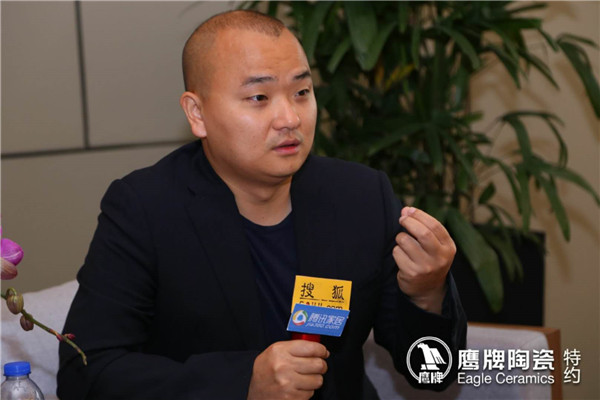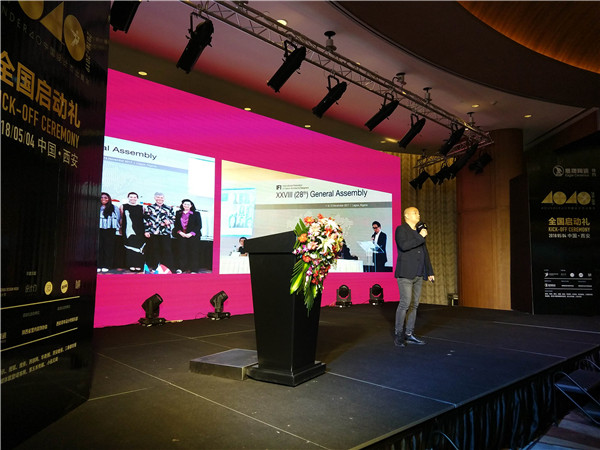|

# 广州设计周执行总监贺文广:保持初心 合作共赢

家装设计，近些年来，得到很多人的关注。而设计师群体，也是一样，受到很多奖项赛事的青睐和诸多媒体的关注。而作为广州设计周执行总监的贺文广先生，对于40UNDER40的设计师评选，他认为有三个关键词：设计力、传播力和执行力可以概括。最近和鹰牌集团的合作，也是让人充满期待的。将设计师和文化传播联系起来

把设计师和文化传播联系起来，这个想法我们大概在四年前，我们去发起做最美瓷砖设计奖项目的时候，当时就是基于这样的初衷去做的一个探索，我们为什么这样去想？ 因为现在当下的瓷砖的设计更多的是在模仿，一个就是模仿所谓的叫自然，石头、木头等天然的东西，第二个就是大量的瓷砖设计几乎是来自于欧美的瓷砖市场，那我们当时为什么一定要去意大利的博洛尼亚展，去学习意大利人、西班牙人的瓷砖设计，因为它的是基于西方人的审美和生活方式和空间尺度去开发和设计的。

那这个一定适合中国人吗？一定符合中国人的生活审美吗？这个不一定。那我们能不能尝试着从中国人的审美、生活方式、空间尺度的需求，来倒推，跟我们的中国的瓷砖企业进行合作。那么谁最了解消费者对于空间的审美需求，随之了解，我们认为是设计师。

我们邀请设计师，因为他们基于当下的中国人的这种生活审美，家装诉求，去创意瓷砖，再叠加我们中国瓷砖的制造企业，把产品给生产出来。这样的话可能会更符合，我们中国的消费者。基于这样的出发点，我们当时做了最美瓷砖奖，当然很荣幸，我们在提出这样的想法的时候，鹰牌集团和华鹏瓷砖，都觉得这个想法非常好，可以一起去做。

设计师跨界做设计，是一种挑战

设计师跨界，我觉得这个是一直以来都有的一个词，不是最近几年才流行的，其实看整个欧美的设计市场，会发现他们现在的建筑设计、室内设计师都在做一个事情，就是产品设计。

为什么会有这样的现象发生，其实就是基于消费者的自主需求越来越多的时候，跟设计师发生最直接的关系就是产品，而产品是最能够和消费者产生共鸣的设计。

所以接下来，我认为中国的建筑设计师也好，室内设计师也好，跨界去做产品的开发，将会变成是一种常态。但是这个常态会有很长一段的预期和那交学费的阶段，为什么这么讲？呢因为整个中国的设计教育体系，在整个过去这几十年的时间，是被切割开的。学建筑设计的跟学室内设计的，跟学产品设计的，大家是在不同的学院，不同的科系成长起来的。因为专业细分，能够让更多的设计师，快速地在专业领域里面就业。但是如果你要去跨界，那他需要去学习的东西会很多。用设计力、传播力、执行力来确保40UNDER40的影响力和号召力

40UNDER40项目，要确保它的影响力和号召力，可以从三个关键词总结来说，第一个关键词我觉得就是叫设计力，设计师能够有影响力，或者能够有号召力的话，那他的专业度是要够的，就是通过评选出来的人或者是他的作品，是具备设计力的，是需要专业度的，在这个板块的话，我们今年会加大专业的投入，40UNDER40跟其他奖项会不太一样，因为我们评选的是人，而且是40岁以下的青年设计师。那我们怎么样去确保评选出来的这个人，他是具备设计力的呢，所以我们会从两个维度去那个评判设计师，第一个是他的作品要好，第二个是他的人品要好，所以我们整个运营体系，可能会跟其他奖项赛事会不太一样。这是第一个，要确保它有设计力，这样的话才会有有影响力。

第二个的话我们叫传播力，任何一个奖项的影响力都是来自于的传播，当然我们希望更多的的媒体加入进来，一起来做40UNDER40这个项目。让更多的设计师能够参与进来，目的其实是为了把好的有设计力的设计师和作品选拔出来，通过我们的传播力传递给更多业主。让消费者能够认识到这批设计师是不错的。也想通过这些传播把设计这个圈子联系起来，让大家在相互的过程当中，把这个圈子在无形当中给扩大，这个过程其实就是大家相互成长、相互学习、相互交流的过程，会获得更大的收益，这个是额外的价值。

第三个我觉得叫执行力，任何一个项目当你讲的天花乱坠，说的特别的高大上，最后都是要靠扎实的执行力来完成的。所以这个也是40UNDER40项目，为什么今年会启动城市榜，我们会落地一百个城市来做城市榜，然后再到30个省区榜，最后再到一个全国榜。

我们就是希望通过这种扎实的落地执行，这种进阶式的方式，让更多的设计师参与进来，让真正优秀的设计师被肯定，然后再最大的舞台上面彰显出来。这个就是我们对40UNDRE40项目的定义，对于如何来确保它的影响力、号召力的思考。

设计驱动设计师成长 设计驱动产业升级 实现美好生活

设计周跟鹰牌的合作渊源追溯起来还蛮深的，我们在创办广州设计周的时候，在2006年我们在做了一个比赛叫金羊奖，当时就是鹰牌参与赞助的，第一年创办的时候，就在跟我们合作，基于跟鹰牌之间的合作，我们跟他们集团三个品牌都在合作，除了鹰牌、鹰牌2086、华鹏都在都在合作。

合作之后，就是对于设计，我们究竟想要干什么？我们想做三个维度，第一个呢我们叫设计驱动设计师的成长。所以就是我们为什么要办大赛？为什么要办论坛？为什么要去做游学？其实都是为了在设计的专业领域里面，先让设计师成长起来，然后成长起来干什么？我们要干的事情叫设计驱动产业升级，刚刚讲的我们把设计师基于文化、基于美感、基于美学，跟我们的瓷砖去进行结合，创造出更好的产品，这个就是在践行设计驱动产业升级的一个具体路径和具体的实施办法之一。

到了第三个维度，设计最终要做的是设计要驱动美好的生活。可能讲的人很多，究竟设计如何来驱动美好的生活？ 其实你会发现前面两个环节做好了，美好生活自动就实现了。我们跟鹰牌这样的国企合作，它相对其他的商业品牌稍微不一样的一点是，它可能真的有那么一点情怀，能和他们产生共鸣。这个是我们跟鹰牌在十几年前我们就开始携手合作，今年又再度携手的一个出发点。

当下的社会环境已经不是一个群体单打独斗的阶段了，其实所有的群体都可以通过某种关系连接起来，就像鹰牌集团、设计周、设计师、中国文化，这四种不同的群体结合起来，会碰撞出什么样的火花，让我们共同期待！

`声明：本文由入驻焦点开放平台的作者撰写，除焦点官方账号外，观点仅代表作者本人，不代表焦点立场错误信息举报电话： 400-099-0099，邮箱：jubao@vip.sohu.com，或点此进行意见反馈，或点此进行举报投诉。`A B C D E F G H J K L M N P Q R S T W X Y Z
A - B - C - D - E
• A
• 鞍山
• 安庆
• 安阳
• 安顺
• 安康
• 澳门
• B
• 北京
• 保定
• 包头
• 巴彦淖尔
• 本溪
• 蚌埠
• 亳州
• 滨州
• 北海
• 百色
• 巴中
• 毕节
• 保山
• 宝鸡
• 白银
• 巴州
• C
• 承德
• 沧州
• 长治
• 赤峰
• 朝阳
• 长春
• 常州
• 滁州
• 池州
• 长沙
• 常德
• 郴州
• 潮州
• 崇左
• 重庆
• 成都
• 楚雄
• 昌都
• 慈溪
• 常熟
• D
• 大同
• 大连
• 丹东
• 大庆
• 东营
• 德州
• 东莞
• 德阳
• 达州
• 大理
• 德宏
• 定西
• 儋州
• 东平
• E
• 鄂尔多斯
• 鄂州
• 恩施
F - G - H - I - J
• F
• 抚顺
• 阜新
• 阜阳
• 福州
• 抚州
• 佛山
• 防城港
• G
• 赣州
• 广州
• 桂林
• 贵港
• 广元
• 广安
• 贵阳
• 固原
• H
• 邯郸
• 衡水
• 呼和浩特
• 呼伦贝尔
• 葫芦岛
• 哈尔滨
• 黑河
• 淮安
• 杭州
• 湖州
• 合肥
• 淮南
• 淮北
• 黄山
• 菏泽
• 鹤壁
• 黄石
• 黄冈
• 衡阳
• 怀化
• 惠州
• 河源
• 贺州
• 河池
• 海口
• 红河
• 汉中
• 海东
• 怀来
• I
• J
• 晋中
• 锦州
• 吉林
• 鸡西
• 佳木斯
• 嘉兴
• 金华
• 景德镇
• 九江
• 吉安
• 济南
• 济宁
• 焦作
• 荆门
• 荆州
• 江门
• 揭阳
• 金昌
• 酒泉
• 嘉峪关
K - L - M - N - P
• K
• 开封
• 昆明
• 昆山
• L
• 廊坊
• 临汾
• 辽阳
• 连云港
• 丽水
• 六安
• 龙岩
• 莱芜
• 临沂
• 聊城
• 洛阳
• 漯河
• 娄底
• 柳州
• 来宾
• 泸州
• 乐山
• 六盘水
• 丽江
• 临沧
• 拉萨
• 林芝
• 兰州
• 陇南
• M
• 牡丹江
• 马鞍山
• 茂名
• 梅州
• 绵阳
• 眉山
• N
• 南京
• 南通
• 宁波
• 南平
• 宁德
• 南昌
• 南阳
• 南宁
• 内江
• 南充
• P
• 盘锦
• 莆田
• 平顶山
• 濮阳
• 攀枝花
• 普洱
• 平凉
Q - R - S - T - W
• Q
• 秦皇岛
• 齐齐哈尔
• 衢州
• 泉州
• 青岛
• 清远
• 钦州
• 黔南
• 曲靖
• 庆阳
• R
• 日照
• 日喀则
• S
• 石家庄
• 沈阳
• 双鸭山
• 绥化
• 上海
• 苏州
• 宿迁
• 绍兴
• 宿州
• 三明
• 上饶
• 三门峡
• 商丘
• 十堰
• 随州
• 邵阳
• 韶关
• 深圳
• 汕头
• 汕尾
• 三亚
• 三沙
• 遂宁
• 山南
• 商洛
• 石嘴山
• T
• 天津
• 唐山
• 太原
• 通辽
• 铁岭
• 泰州
• 台州
• 铜陵
• 泰安
• 铜仁
• 铜川
• 天水
• 天门
• W
• 乌海
• 乌兰察布
• 无锡
• 温州
• 芜湖
• 潍坊
• 威海
• 武汉
• 梧州
• 渭南
• 武威
• 吴忠
• 乌鲁木齐
X - Y - Z
• X
• 邢台
• 徐州
• 宣城
• 厦门
• 新乡
• 许昌
• 信阳
• 襄阳
• 孝感
• 咸宁
• 湘潭
• 湘西
• 西双版纳
• 西安
• 咸阳
• 西宁
• 仙桃
• 西昌
• Y
• 运城
• 营口
• 盐城
• 扬州
• 鹰潭
• 宜春
• 烟台
• 宜昌
• 岳阳
• 益阳
• 永州
• 阳江
• 云浮
• 玉林
• 宜宾
• 雅安
• 玉溪
• 延安
• 榆林
• 银川
• Z
• 张家口
• 镇江
• 舟山
• 漳州
• 淄博
• 枣庄
• 郑州
• 周口
• 驻马店
• 株洲
• 张家界
• 珠海
• 湛江
• 肇庆
• 中山
• 自贡
• 资阳
• 遵义
• 昭通
• 张掖
• 中卫

1室1厅1厨1卫1阳台

1
2
3
4
5

0
1
2

1

1

0
1
2
3报名成功，资料已提交审核A B C D E F G H J K L M N P Q R S T W X Y Z
A - B - C - D - E
• A
• 鞍山
• 安庆
• 安阳
• 安顺
• 安康
• 澳门
• B
• 北京
• 保定
• 包头
• 巴彦淖尔
• 本溪
• 蚌埠
• 亳州
• 滨州
• 北海
• 百色
• 巴中
• 毕节
• 保山
• 宝鸡
• 白银
• 巴州
• C
• 承德
• 沧州
• 长治
• 赤峰
• 朝阳
• 长春
• 常州
• 滁州
• 池州
• 长沙
• 常德
• 郴州
• 潮州
• 崇左
• 重庆
• 成都
• 楚雄
• 昌都
• 慈溪
• 常熟
• D
• 大同
• 大连
• 丹东
• 大庆
• 东营
• 德州
• 东莞
• 德阳
• 达州
• 大理
• 德宏
• 定西
• 儋州
• 东平
• E
• 鄂尔多斯
• 鄂州
• 恩施
F - G - H - I - J
• F
• 抚顺
• 阜新
• 阜阳
• 福州
• 抚州
• 佛山
• 防城港
• G
• 赣州
• 广州
• 桂林
• 贵港
• 广元
• 广安
• 贵阳
• 固原
• H
• 邯郸
• 衡水
• 呼和浩特
• 呼伦贝尔
• 葫芦岛
• 哈尔滨
• 黑河
• 淮安
• 杭州
• 湖州
• 合肥
• 淮南
• 淮北
• 黄山
• 菏泽
• 鹤壁
• 黄石
• 黄冈
• 衡阳
• 怀化
• 惠州
• 河源
• 贺州
• 河池
• 海口
• 红河
• 汉中
• 海东
• 怀来
• I
• J
• 晋中
• 锦州
• 吉林
• 鸡西
• 佳木斯
• 嘉兴
• 金华
• 景德镇
• 九江
• 吉安
• 济南
• 济宁
• 焦作
• 荆门
• 荆州
• 江门
• 揭阳
• 金昌
• 酒泉
• 嘉峪关
K - L - M - N - P
• K
• 开封
• 昆明
• 昆山
• L
• 廊坊
• 临汾
• 辽阳
• 连云港
• 丽水
• 六安
• 龙岩
• 莱芜
• 临沂
• 聊城
• 洛阳
• 漯河
• 娄底
• 柳州
• 来宾
• 泸州
• 乐山
• 六盘水
• 丽江
• 临沧
• 拉萨
• 林芝
• 兰州
• 陇南
• M
• 牡丹江
• 马鞍山
• 茂名
• 梅州
• 绵阳
• 眉山
• N
• 南京
• 南通
• 宁波
• 南平
• 宁德
• 南昌
• 南阳
• 南宁
• 内江
• 南充
• P
• 盘锦
• 莆田
• 平顶山
• 濮阳
• 攀枝花
• 普洱
• 平凉
Q - R - S - T - W
• Q
• 秦皇岛
• 齐齐哈尔
• 衢州
• 泉州
• 青岛
• 清远
• 钦州
• 黔南
• 曲靖
• 庆阳
• R
• 日照
• 日喀则
• S
• 石家庄
• 沈阳
• 双鸭山
• 绥化
• 上海
• 苏州
• 宿迁
• 绍兴
• 宿州
• 三明
• 上饶
• 三门峡
• 商丘
• 十堰
• 随州
• 邵阳
• 韶关
• 深圳
• 汕头
• 汕尾
• 三亚
• 三沙
• 遂宁
• 山南
• 商洛
• 石嘴山
• T
• 天津
• 唐山
• 太原
• 通辽
• 铁岭
• 泰州
• 台州
• 铜陵
• 泰安
• 铜仁
• 铜川
• 天水
• 天门
• W
• 乌海
• 乌兰察布
• 无锡
• 温州
• 芜湖
• 潍坊
• 威海
• 武汉
• 梧州
• 渭南
• 武威
• 吴忠
• 乌鲁木齐
X - Y - Z
• X
• 邢台
• 徐州
• 宣城
• 厦门
• 新乡
• 许昌
• 信阳
• 襄阳
• 孝感
• 咸宁
• 湘潭
• 湘西
• 西双版纳
• 西安
• 咸阳
• 西宁
• 仙桃
• 西昌
• Y
• 运城
• 营口
• 盐城
• 扬州
• 鹰潭
• 宜春
• 烟台
• 宜昌
• 岳阳
• 益阳
• 永州
• 阳江
• 云浮
• 玉林
• 宜宾
• 雅安
• 玉溪
• 延安
• 榆林
• 银川
• Z
• 张家口
• 镇江
• 舟山
• 漳州
• 淄博
• 枣庄
• 郑州
• 周口
• 驻马店
• 株洲
• 张家界
• 珠海
• 湛江
• 肇庆
• 中山
• 自贡
• 资阳
• 遵义
• 昭通
• 张掖
• 中卫• 手机• 分享
• 设计
免费设计
• 计算器
装修计算器
• 入驻
合作入驻
• 联系
联系我们
• 置顶
返回顶部